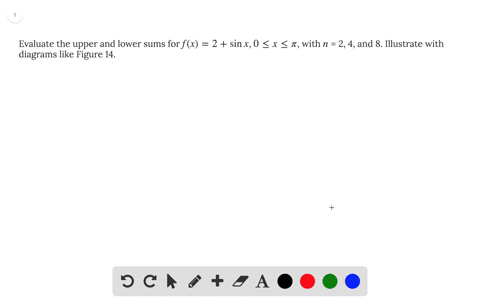## Discussion

You must be signed in to discuss.

## Video Transcript

Yeah, so is usually leaves a graphic part for you. Use a graft calculations to do that. And I'll tell you how to r right how's s made in terms ofthe f by using those endpoint method. So, for example, the first part of the problem as us too estimates area under the F by using for approximately in rectangles and our access from one, two, five. So how to do that? If we use for our tangos, then then the point. I exactly one to five. So if we are using the left end point, for example Sorry. In this case, they asked us to use right in point. So, for example, values in a right in point. Then we are taking the height of the rectangle will be the functions of area at this point. This point this point at this point, and the base of it shook him a little bit. What? So for examples of be part, what asked me who is right on point with a fork approximately rectangle On's estimate will be the base of the rectangle times the sum of height which will be F off too, plus f off three plus four plus F five and have a middle point. Well, the concept is similar if we if we use middle point matter, that means the height of your tango appease a functional value at those points and the face of his truck tango is still what that means. That means he fool us. Middle point. Then the estimate will be based on the rectangles times the height. Take a look. Assemble point Should be point five for so one point by two point vice three point five and four point five. So with some hoes high together So you half off one point five plus f off two point five Step off three point five Plus there for four point five Again f off those value You have the formula off the function Then I'll leave you to use a calculator to figure out those values. Improve the estimate by using direct on Rose. So here you go from one to five. This is a middle point for for a tinkle. So now if we was a truck goes from one to five Try try here. You don't have Detroit. If they ask you to use many rectangles, you just have to know. Like, for example, Zeiss Room can go to the left and point Ryan for here. So here we have, and I'm not going right point five paces. You have idea. This is point one point five two point five for five. If we used a trick tango and use a Ryan point What we can't. If we used right in point, then the height of the rectangles will be the function value at those points. So? So the area estimates off using the Ryan point Matthew will be the base of Israel. Fell in this case will be one over to a point five times of some of the height of a rectangle which are so value of the functions off at those points so f off one point five. Plus it off to us. They're for four point five plus f Fine. I have a middle point. Sit. Oh, except that now we're using those point us. Like the function value at those points as the height of the rectangle. Uh, the base of the structure most still point five. And we used those green point us as a symbol for it. And we take the Heidelberg tango us the value of the function at those points. So, for example, the first one will be half off one point two five. The second one will be air for four point seven five and the last one will be air for four point seven five. And again you can probably those number into into the given function FX. It was X minus two national oh, cracks and pulling to the calculator to figure out PNR number Here, I tell you how to write a formal outfit.